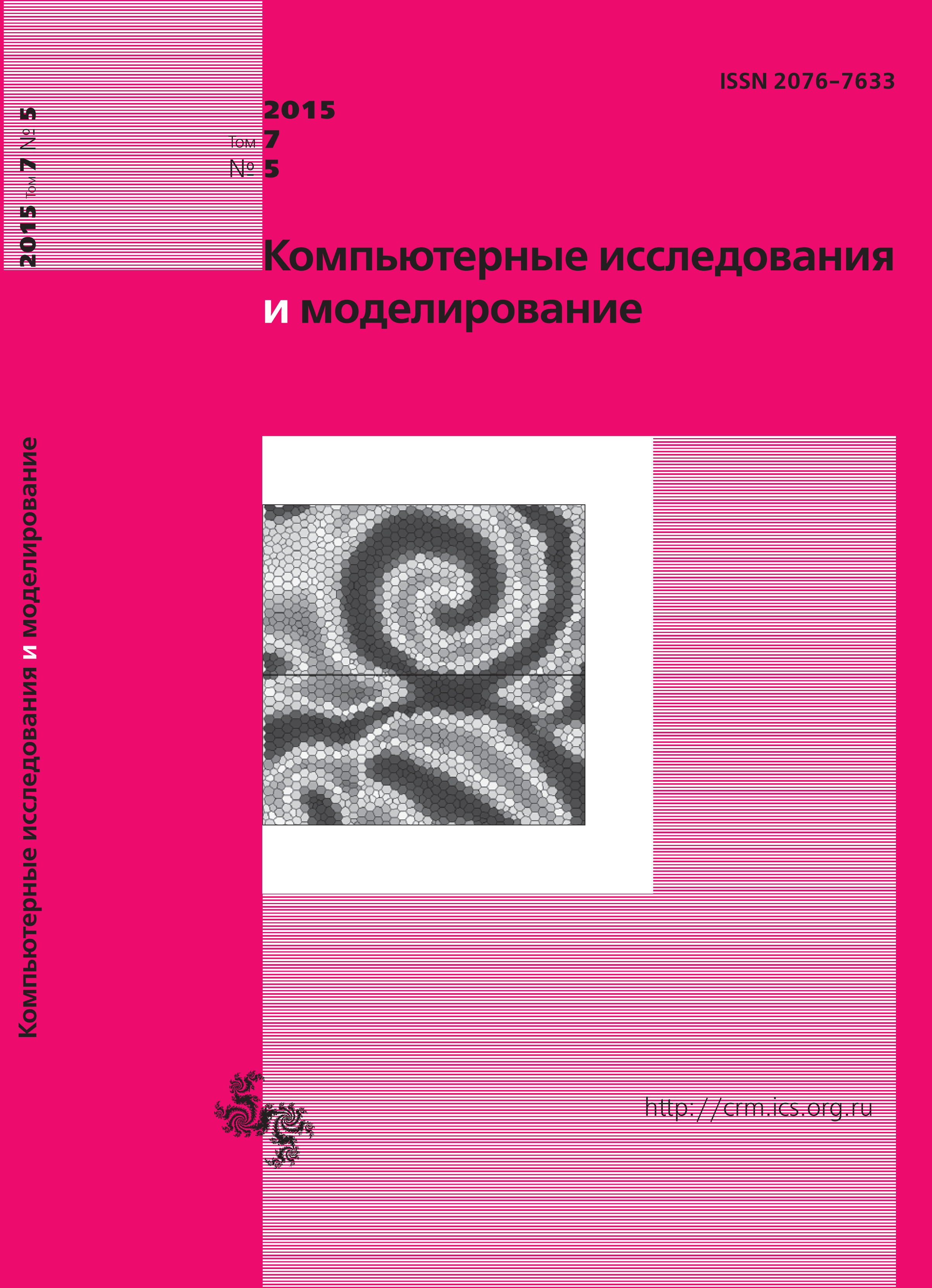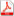Issue 5, 2015 Vol. 7

# All issues

Mathematical modeling of bending of a circular plate using $S$-splinespdf (220K)  / List of references

This article is dedicated to the use of higher degree $S$-splines for solving equations of the elasticity theory. As an example we consider the solution to the equation of bending of a plate on a circle. $S$-spline is a piecewise-polynomial function. Its coefficients are defined by two conditions. The first part of the coefficients are defined by the smoothness of the spline. The rest are determined using the least-squares method. We consider class $C^4$ 7th degree $S$-splines.

Keywords: approximation, spline, numerical methods, method of finite elements, the mathematical physics, the elasticity theory
Citation in English: Fedosova A.N., Silaev D.A. Mathematical modeling of bending of a circular plate using $S$-splines // Computer Research and Modeling, 2015, vol. 7, no. 5, pp. 977-988
Citation in English: Fedosova A.N., Silaev D.A. Mathematical modeling of bending of a circular plate using $S$-splines // Computer Research and Modeling, 2015, vol. 7, no. 5, pp. 977-988
DOI: 10.20537/2076-7633-2015-7-5-977-988
Views (last year): 4.

Full-text version of the journal is also available on the web site of the scientific electronic library eLIBRARY.RU

The journal is included in the Russian Science Citation Index

The journal is included in the List of Russian peer-reviewed journals publishing the main research results of PhD and doctoral dissertations.

International Interdisciplinary Conference "Mathematics. Computing. Education"

The journal is included in the RSCI

Indexed in Scopus